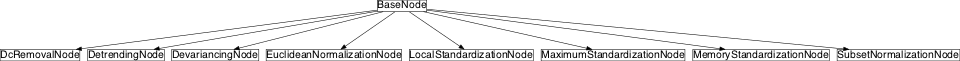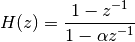# normalization¶

## Module: missions.nodes.preprocessing.normalization¶

Normalize TimeSeries channel-wise

Normalize can mean to translate the values of each channel so that its mean becomes zero and/or to scale each channels values so that the standard deviation becomes 1.

Inheritance diagram for pySPACE.missions.nodes.preprocessing.normalization:## Class Summary¶

 DetrendingNode([detrend_method, ...]) Eliminate trend on the selected channels with the given function (default: mean) LocalStandardizationNode(\*\*kwargs) Z-Score transformation (zero mean, variance of one) MaximumStandardizationNode(\*\*kwargs) Standardize by subtracting the mean and dividing by maximum value MemoryStandardizationNode([order]) Z-Score transformation with respect to the last order windows DevariancingNode(devariance_method[, ...]) Apply devariancing method on training data and use the result for scaling SubsetNormalizationNode(subset[, devariance]) Z-Score transformation by the mean and variance of a subset of the samples EuclideanNormalizationNode([selected_channels]) Scale all channels to norm one DcRemovalNode([alpha, selected_channels]) Perform a realtime DC removal on the selected channels

## Function Summary¶

 check_zero_division(self, data[, tolerance, ...]) Increase to small values in data and give warning

## Classes¶

### DetrendingNode¶

class pySPACE.missions.nodes.preprocessing.normalization.DetrendingNode(detrend_method=<function detrend_mean>, selected_channels=None, **kwargs)[source]

Eliminate trend on the selected channels with the given function (default: mean)

This method takes one function that is used for detrending of the selected channels of each time series instance that is passed through this node (e.g. the matplotlib.mlab.detrend_mean function).

Parameters
detrend_method: selected_channels: Method being applied to each selected channel, to detrend. (optional, default: “eval(__import__(‘pylab’).detrend_mean)”) List of channel names, were the method is applied to (optional, default: None = all channels)

Exemplary Call

-
node : Detrending

Authors: Created: Jan Hendrik Metzen (jhm@informatik.uni-bremen.de) 2009/01/06 Detrending DetrendingNode TimeSeries

Class Components Summary

 _execute(data) Apply the detrending method to the given data x and return a new time series input_types
__init__(detrend_method=<function detrend_mean>, selected_channels=None, **kwargs)[source]
_execute(data)[source]

Apply the detrending method to the given data x and return a new time series

input_types = ['TimeSeries']

### LocalStandardizationNode¶

class pySPACE.missions.nodes.preprocessing.normalization.LocalStandardizationNode(**kwargs)[source]

Z-Score transformation (zero mean, variance of one)

Each channel of the resulted multidimensional time series has a mean of 0 and a standard deviation of 1.

Parameters

Exemplary Call

-
node : Standardization

Author: POSSIBLE NODE NAMES: Anett Seeland (Anett.Seeland@dfki.de) LocalStandardization LocalStandardizationNode Standardization TimeSeries

Class Components Summary

 _execute(x) Apply z-score transformation to the given data and return a modified time series. input_types
input_types = ['TimeSeries']
__init__(**kwargs)[source]
_execute(x)[source]

Apply z-score transformation to the given data and return a modified time series.

### MaximumStandardizationNode¶

class pySPACE.missions.nodes.preprocessing.normalization.MaximumStandardizationNode(**kwargs)[source]

Standardize by subtracting the mean and dividing by maximum value

Each channel of the resulted multidimensional time series has a mean of 0 and the maximum value will be 1.

Parameters

Exemplary Call

-
node : MaximumStandardization

Authors: POSSIBLE NODE NAMES: Hendrik Woehrle (hendrik.woehrle@dfki.de), Yohannes Kassahun (kassahun@informatik.uni-bremen.de) MaximumStandardization MaximumStandardizationNode TimeSeries

Class Components Summary

__init__(**kwargs)[source]
_execute(x)[source]
input_types = ['TimeSeries']

### MemoryStandardizationNode¶

class pySPACE.missions.nodes.preprocessing.normalization.MemoryStandardizationNode(order=0, **kwargs)[source]

Z-Score transformation with respect to the last order windows

mean = 1/(order+1) sum_{i=0}^{order} mean[time_{-i}] std = sqrt(1/(order+1) sum_{i=0}^{order} var[time_{-i}])

If the standard deviation is to high because of artifacts, we only use the current standard deviation and it is not put into memory.

Parameters
order: Number of previously occurred time windows being considered for calculation of standard deviation and mean (optional, default: 0)

Exemplary Call

-
node : Memory_Standardization
parameters:
order : 3

Author: POSSIBLE NODE NAMES: Mario Krell (Mario.Krell@dfki.de) MemoryStandardization MemoryStandardizationNode Memory_Standardization TimeSeries

Class Components Summary

 _execute(x) Apply memory z-score transformation to the given data and return a new time series. input_types
__init__(order=0, **kwargs)[source]
_execute(x)[source]

Apply memory z-score transformation to the given data and return a new time series.

input_types = ['TimeSeries']

### DevariancingNode¶

class pySPACE.missions.nodes.preprocessing.normalization.DevariancingNode(devariance_method, selected_channels=None, **kwargs)[source]

Apply devariancing method on training data and use the result for scaling

This method takes one function that is used for devariancing of the selected channels of the whole data set (e.g. a scaling the channels so that they have all standard deviation 1).

Note

The scaling factors are calculated on a training set and remain equal over the whole execution time. They do not change with each window.

Parameters
devariance_method:

Method being applied to each selected channel of the signal, to devariance.

selected_channels:

List of channel names, were the method is applied to. If nothing is specified, all channels are used.

(optional, default: None)

Exemplary Call

-
node : Devariancing
parameters :
devariance_method : eval(__import__('numpy').std)

Authors: Created: Jan Hendrik Metzen (jhm@informatik.uni-bremen.de) 2009/01/06 DevariancingNode Devariancing TimeSeries

Class Components Summary

 _execute(x) Apply devariancing to the given data and return the modified time series _stop_training() Uses the devariance method to determine scaling factors for each _train(x) The node gathers all data point of the selected channels input_types is_trainable() Returns whether this node is trainable
__init__(devariance_method, selected_channels=None, **kwargs)[source]
is_trainable()[source]

Returns whether this node is trainable

_train(x)[source]

The node gathers all data point of the selected channels during training

_stop_training()[source]

Uses the devariance method to determine scaling factors for each selected channel

_execute(x)[source]

Apply devariancing to the given data and return the modified time series

input_types = ['TimeSeries']

### SubsetNormalizationNode¶

class pySPACE.missions.nodes.preprocessing.normalization.SubsetNormalizationNode(subset, devariance=False, **kwargs)[source]

Z-Score transformation by the mean and variance of a subset of the samples

Thus, in LRP scenarios, e.g., one could perform a shift driven by the first x samples of the window. This would arrange the “baseline”, such that “pre LRP” is zero. In EEG data processing, this procedure is commonly referred to as “baseline correction”

The resulting TimeSeries are not regularized in the sense that they are not in (0,1).

Parameters
subset: List of samples being used to calculate the mean. Also divide by the variance (optional, default: False)

Exemplary Call

-
node : SubsetNormalization
parameters :
subset : eval(range(4)) # or any list

Author: POSSIBLE NODE NAMES: Mario Krell (mario.krell@dfki.de) SubsetNormalization SubsetNormalizationNode TimeSeries

Class Components Summary

 _execute(data) Perform a shift and normalization according input_types
__init__(subset, devariance=False, **kwargs)[source]
_execute(data)[source]

Perform a shift and normalization according (whole_data - mean(specific_samples)) / std(specific_samples)

input_types = ['TimeSeries']

### EuclideanNormalizationNode¶

class pySPACE.missions.nodes.preprocessing.normalization.EuclideanNormalizationNode(selected_channels=None, **kwargs)[source]

Scale all channels to norm one

Together with detrending this is equivalent to standardization.

Exemplary Call

-
node : Euclidian_Feature_Normalization

Authors: Created: Hendrik Woehrle (hendrik.woehrle@dfki.de) 2011/07/28 Euclidian_Feature_Normalization EuclideanNormalization EuclideanNormalizationNode TimeSeries

Class Components Summary

 _execute(x) Apply the detrending method to the given data x and return a new time series. input_types
__init__(selected_channels=None, **kwargs)[source]
_execute(x)[source]

Apply the detrending method to the given data x and return a new time series.

input_types = ['TimeSeries']

### DcRemovalNode¶

class pySPACE.missions.nodes.preprocessing.normalization.DcRemovalNode(alpha=0.95, selected_channels=None, **kwargs)[source]

Perform a realtime DC removal on the selected channels

A node that uses the realtime DC removal method by IIR filtering withThe filters internal state is preserved between blocks.

For details:

R. Lyons, “Understanding Digital Signal Processing”, 2nd ed., 13.23.2 Real-Time DC Removal, p.553

Parameters

alpha: selected_channels: See parameter in formula List of channel names, were the method is applied to (optional, default: None = all channels)

Exemplary Call

-
node : DcRemoval
parameters :
alpha : 0.95

Authors: Created: Hendrik Woehrle (hendrik.woehrle@dfki.de) 2011/08/18 Dc_Removal DcRemoval DcRemovalNode TimeSeries

Class Components Summary

 _execute(data) Apply the scipy.signal.lfilter() function with the DC removal coefficients on the data input_types
input_types = ['TimeSeries']
__init__(alpha=0.95, selected_channels=None, **kwargs)[source]
_execute(data)[source]

Apply the scipy.signal.lfilter() function with the DC removal coefficients on the data

## check_zero_division()¶

pySPACE.missions.nodes.preprocessing.normalization.check_zero_division(self, data, tolerance=1e-15, data_ts=None)[source]

Increase to small values in data and give warning

This global function of this module checks whether numpy array ‘data’ contains values below the specified ‘tolerance’. If so, the value of 1 is added to this value and a warning is put into the log file. Returned is the modified array.

Parameters
self: Needs the node object for proper logging. Numpy array which is checked for values below certain tolerance. Values in data below this value are corrected. (optional, default: 10^-9) Actual TimeSeries object to improve information in logging. If not specified, the logging message is simplified. (optional, default: None)